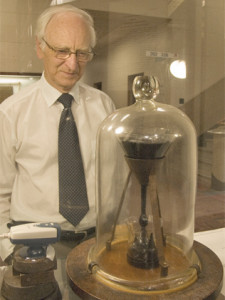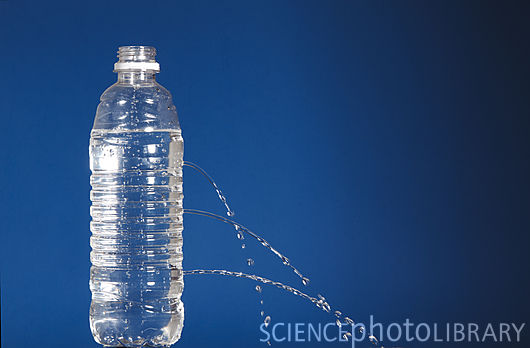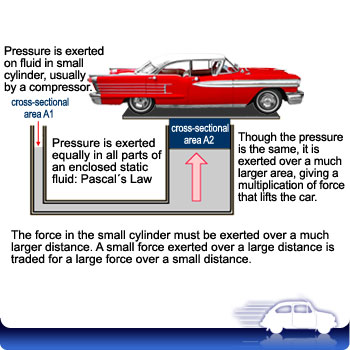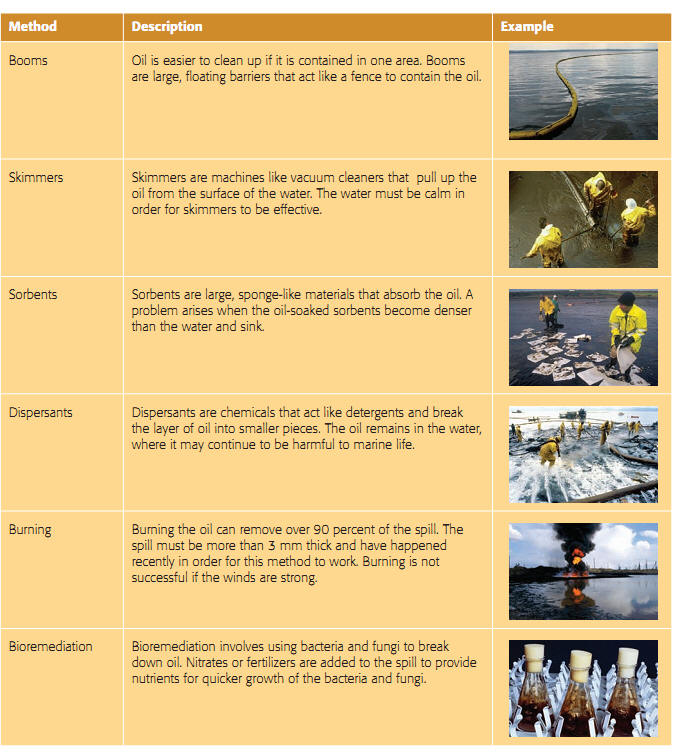# Fluids

What is a Fluid?

A fluid is a substance that has the ability to flow. This means that both liquids and gasses are fluids even though in everyday speech we often use the word fluid when we mean to say liquid. Fluids do a great many things for us and we use them in a variety of circumstances. They can hold dissolved substances, like the cytoplasm in our cells, or sugars dissolved in our soft drinks and they can carry solid particles in slurries. Fluids can also become solids and we often make use of this when we control the exact shape into which a fluids solidifies, like for example when we pour concrete and let it harden or in 3D printing. What counts as a fluid and what doesn’t isn’t always easy to determine. Some fluids flow so slowly (hyper-viscous fluids) that it is not possible to observe the actual flowing. There is a famous experiment with a hyper-viscous fluid (pitch) that takes on average 10 years for a single drop to fall off the main fluid body.
http://en.wikipedia.org/wiki/Pitch_drop_experimentFluids and the Particle Theory:

The defining ability of a fluid is its ability to flow and allow passage of other materials through it. Again, Particle Theory is helpful in understanding this situation. Unlike in a solid, in liquids and especially in gasses, the bonds that hold particles together are weak or pretty much absent. Thus, particles are free to a greater and greater degree to move around, exchange place and outright leave the cohesive lattice of connections (in gasses). The lower the attractive forces (bonds) between particles, the better the fluid can flow, which brings us to our next point.
Viscosity:

The ability of a fluid to flow varies with a number of factors but primarily depends on the strength of the bonds between particles. Thus, the weaker the bonds, the better a fluid flows. We call this ability to flow the flow rate. Here now comes the tricky part, viscosity measures the opposite of flow rate, it measures the resistance to flowing, in other words how badly a fluid flows. In reality it measures the internal friction in a fluid but it’s ok to just look at it from a perspective of how well ( actually how “not well”) a fluid flows.

A fluid with high viscosity flows slowly/ poorly. Think tar, cold honey or molasses.

A fluid with low viscosity flows well/ quickly. Think water, hot oil or any gas.

We say that flow rate and viscosity are inversely proportional, meaning that as one increases, the other decreases by a predictable and proportional amount.

Factors that Affect Viscosity:

As mentioned earlier, the chemical and atomic nature of a substance has a major influence on its viscosity because it determines the type and number or bonds that form between particles. Stronger bonds mean a lesser degree of freedom and thus a slower flow rate/higher viscosity. Other factors are:

Temperature:

Higher temperature in liquids weaken bonds and thus decrease viscosity. Things are different in gasses since gas particles have pretty much no bonds and thus these bonds cannot be weakened (nonexistence has that effect on things). So what happens in gasses? Well, gasses generally have very low viscosities to start with. The little bit of viscosity in gasses is due to getting into each other way by collisions. Higher temperatures tend to increase the number of collisions and thus higher temperatures in gasses tend to increase viscosity.

Particle Structure:

If all other factors are equal, the more “structurally awkward and complex” a particle is the more it will interfere with particle flow and thus increase viscosity. Thus, branchy particles tend to flow less well than linear particles (straight line structure).

This video is a 3 year timelapse of the famous pitch drop experiment from the University of Queensland, Australia. Pitch is a substance that challenges our view of what a fluid is. It flows so slowly, that, under airconditioned temperatures, a single drop takes more than a decade to drop and detach completely from the main body.

Density:

Density measure how tightly packed particles are in a substance. More precisely, it measures how much mass there is in a given volume so both the packing as well as the atomic weight of the individual particles matter.
It is measured in mass per volume    Density = Mass/Volume

For example: Say we have a substance that has a mass of 500g and occupies a volume of 125 ml. Its density then would be 500g/125ml= 4g/ml
By comparison, the density of water is 1g/ml

Here is the triangle for this equation.1) What is the density of a material if 400g of this substance have a volume of 2000ml?

2) What is the density of a material if 2500g of this material have a volume of 500ml?

3) What is the volume of 450g of lead if lead has a density of 11.3 g/ml?

4) What is the mass of 1200ml of copper if copper has a density of 8.9 g/ml

We can determine if something will float or sink in a given fluid by comparing densities:

If the density of a substance is greater than the density of the fluid, it will sink.

If the density of a substance is lower than that of the fluid, it will float.

If the density of a substance is the same as that of the fluid it will neither sink nor float but “hover”.

Density is affected by temperature. Remember that a rise in temperature tends to expand objects, but the mass of the object stays the same (I hope that is obvious). So if we increase volume without increasing mass in our Density = mass/volume calculation it should be easy to see that our density value is reduced by increases in temperature. Density tends to decrease as temperature increases.
Let’s do an example:

Our example above was as 500g object with a volume of 15 ml.
density = 500g/125ml = 4g/ml

Let’s say an increase of temperature comes with a 20% volume increase. The object now has a volume of 150ml instead of 125 ml. Let’s do the calculation again with our new value.

Density = 500g/150ml = 3.33g/ml
Our density has decreased

These last two bits of information mean that we should expect hot fluids to rise, which is exactly what we see. How air rises and warmer water floats on top of cooler water (take a swim in a lake during the summer if you don’t believe me. I’m assuming you know how to swim.)

Buoyancy:

Buoyancy is what an object experiences when it is partially or completely submerged in a fluid. When an object enters the fluid the fluid particles move out of the way to make room for the object. What actually happens is a bit complicated but the end effect basically is that the fluid “pushes back” of the object. The more fluid is displaced (pushed out of the way), in other words the more volume of fluid is displaced, the greater the resulting force called the buoyant force on the object. The buoyant force pushes back exactly opposite to gravity (there’s a good reason for that but that’ll have to wait). In fact, the buoyant force on the object is exactly equal to the weight of the fluid the object displaces. This is known as Archimedes’ Principle.
Let’s think this through. Basically the more volume the object has the more volume of fluid it could potentially displace and the weight of that fluid (ultimately its mass) determines the buoyant force on the object. The gravitational force on the object also is a function of its mass so the two forces that are in competition here (buoyancy and gravity) will determine if an object will float, sink or hover. Ultimately all this can be reduced to comparing the densities of object and fluid. The less dense the object the more fluid it can displace and the greater buoyancy is, the denser the fluid is, the greater the weight of that displaced fluid is and the greater buoyancy will be.

Let’s examine an actual case:

Let’s take our 4g/ml object from before. It has a mass of 500g and a volume of 125 ml. This means that the maximum fluid it can displace if 125ml, it’s total volume.
If we put this block into water (density 1g/ml) that means that 125ml of water can be displace which is the equivalent of 125g of water. So we have 500g of object being pulled down and only 125g of water pushing back. This object will sink.

However, If we put the object into liquid mercury (density of 13.55g/ml) the situation changes.
At total submersion the object continues to displace 125ml, but 125ml of mercury have an equivalent mass of 125ml*13.55g/ml = 1693.75 g
This object displaces more than 1.5kg of mercury but it would only take 0.5kg to make it hover. As a matter of fact it would only take a fraction of it’s volume to make it float.
See if you can figure out how far the object would sink into the mercury.

Pressure:

Pressure is the amount of force applied to a certain area. Pressure = Force/Area

P = F/A

There are a number of pressure measurements out there but they all work in this way; some force is spread over some measure of area.
For example, the metric measurement of force is the Pascal (Pa) and is reached by using the metric measurements of force and area:

1Pascal = 1Newton/ 1 Meter2

This is the equivalent of a mass of 100g in Earth’s gravitational field spread over a 1meter by 1 meter area. The Pascal is a small unit, like the second or the Joule. For example, atmospheric air pressure ranges somewhere around the 100,000 Pa (or 100 kPa).
Pressure and Depth:

In a gravitational field, like the one we’re in right now on Earth, pressure in a fluid increases with depth. The deeper in a fluid we are, the greater the column of fluid is that presses down on us due to gravity. A greater column exerts a greater force. An illustration of this can be seen when we poke holes at varying heights in a water bottle. the resulting streams of water from the bottle will vary with intensity and thus distance depending on pressure. Greater pressure results in the stream being pushed farther away from the bottle.Liquid pressure. In a gravitational field liquid pressure increases with depth. Here, a plastic bottle has three small holes on its side. The speed of water flowing through the holes increases with depth because of the increasing liquid pressure.

Pressure and Fluid Flow

Pressure is the result of the application of force. Objects and materials are moved by forces if the forces are large enough and the object or material has “somewhere to move to”. It should come as no surprise then that fluids will flow as a result of pressure application if they have somewhere to flow to. Fluids flow from high to low pressure areas. “Sucking” on a straw, for example, creates a low pressure area in your mouth and atmospheric pressure pushes the liquid, from a frappuccino say, into your mouth.

Compression

When a force is applied to an object it may shrink in volume as a result. We call this decrease in volume in response to a force compression. In a gas, particles are far apart and thus they can respond to an applied force by moving closer together. Gasses are thus said to be compressible. Liquids are a different story. The disorganized arrangement of particles in a liquid with bonds strong enough to keep the volume constant means that particles are pretty much as tightly packed as they can be. This means that particles in a liquid have nowhere to go as a response to an external force and thus liquids do not compress well, or pretty much not at all. Liquids are said to be incompressible. For both liquids and gasses though, external forces increase pressure in the fluid, which leads us to our next big concept:
Pascal’s Law

Since the particles in a fluid are free to move around, any force that is applied to an enclosed fluid is spread equally in that fluid. Properly stated, this is Pascal’s Law:
When a force is applied to an enclosed fluid, the increase in pressure is transmitted equally to all parts of the fluid.

Enclosed fluid systems are thus able to transmit a force to all parts of the system. We make use of this principle in Hydraulic (enclosed liquid systems) and Pneumatic (enclosed gas systems) systems.

We also combine this principle with the principle of mechanical advantage. An enclosed fluid system can multiply our force if we use different size pistons at the input and output end. Since the pressure is equal at both ends, a larger piston has a larger area and thus produces a larger force. The trade off, as always, is that the input piston must travel a distance that is larger by the same factor by which the system increases the force. A doubling of force means the input piston must travel twice as far.Fluid Spills and Contamination

Let’s switch gear a little. One of the most useful traits of fluids is that they can carry other substances over great distances and for a long time. When that substance is not desirable though, we have a problem.

Oil is less dense than water and thus floats on top of it. Oil is not a healthy substance to be around; it has many toxic components, prevents gas exchange, reduces the insulating ability of fur and feathers and kills buoyancy of sea birds.

Oil enters our fluid systems through a variety of processes:

•  routine maintenance
• big spills
• offshore drilling
• burning
• commercial and domestic drains
• natural seeps

Many other contaminants routinely enter our air and water systems. Such as:

•  Pharmaceuticals
•  Industrial waste
•  Sewage
•  Farm runoff
•  Pesticides
•  Vehicle exhausts

Cleaning up Oil SpillsThis leads us to our last unit on Water Systems 🙂